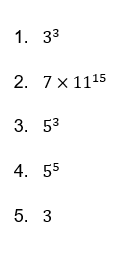# Exponents and Powers Worksheet (with answer key)

The result of multiplying a number by itself is power. Typically, a base number and an exponent are used to represent power. What number is being multiplied is revealed by the base number. A small number that is written above and to the right of the base number, called the exponent, indicates how many times the base number has been multiplied.

What is the “Exponents and Powers Worksheet (with answers)”?

This worksheet will explore the Exponents and Powers Worksheet.

## What are the Exponents and Powers Worksheet?

Exponents and powers can be used to represent extremely large or extremely small numbers in a more straightforward way. It had laws and rules.

This worksheet will help you solve and be more familiar with the laws and rules of exponents.

Instructions on how to use the “Exponents and Powers Worksheet (with answers).”

Study the idea and the provided examples, then attempt to complete the exercises below.

## Conclusion

Exponents are used to raise a number to a power, with the exponent serving as the power. The base of power is the number it is raised to; in the case of the number, 23, the base is 2 and the exponent is 3. The exponent is the power raised to the base number, whereas the power is represented as the combination of the base number and the exponent.

If you have any inquiries or feedback, please let us know.

## Exponents and Powers Worksheet (with answer key)

An exponent of a number represents the number of times the number is multiplied by itself.

The result of multiplying 4 by itself n times is represented as follows: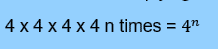The above expression, 4n, is pronounced as 4 raised to the power n. Therefore, exponents are also sometimes referred to as power or indices.

There are exponent rules or laws of exponents.

According to this rule, an exponential number whose exponent is the sum of the exponents of the two numbers is produced when two exponential numbers with the same base but different exponents are added together.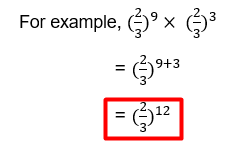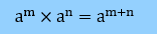According to this rule, an exponential number whose exponents are the difference between the exponents of the two numbers is the quotient of two exponential numbers with the same base but different exponents.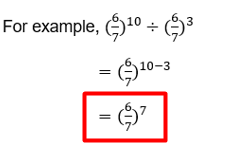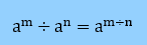According to this rule, the base is raised to the product of the two products after the exponential number is raised to a power.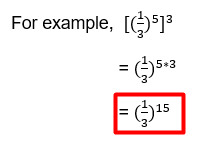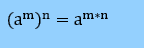## Worksheet

1. Simplify and express each of the following in exponential form.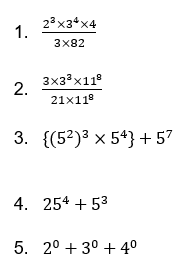1. Reflection

What do you think about solving the Exponents and Powers? Did you find it difficult to follow the pattern in each step?